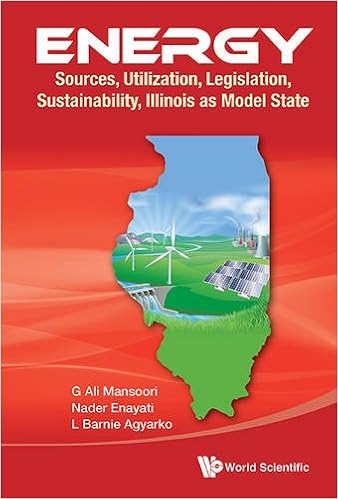## Equations of State

The Hard-Sphere Based Cubic Equation of State
KU-ChE Reactor Journal, 2(3): pp. 2-9, 2008.
A cubic hard-core equation of state
Fluid Phase Equilibria, Volume 206, No.s 1-2, pp.27-39, 30 April 2003.
New equation of state is accurate for VLE, PVT calculations of oil mixtures
Oil & Gas Journal, December 9, 2002.
An equation of state for property prediction of alcohol - hydrocarbon and water-hydrocarbon systems
J. Petrol. Sci. & Eng., Vol.32, pp.103-114, 2001.
Thermodynamic behavior of hydrogen / natural gas mixtures
Proceedings of the 74th Annual Convention of the Gas Processors Association, GPA, Tulsa, OK, pp.23-27, 1995.
A cubic equation of state based on a simplified hard-core model
Chem. Eng. Comm. Vol. 131, pp.15-31, 1995.
Sour natural gas and liquid equation of state
J. Petrol. Science & Engineering, Vol. 12, pp.127-136, 1994.
Molecular based cubic equations of state
Scientia Ir., Vol. 1, No. 3, pp.193-204, 1994.
Simple equation of state accurately predicts hydrocarbon densities
Oil & Gas Journal, pp.108-111, July 12, 1993.
Use of Velocity of Sound in Predicting PVT Relations of Dense Fluids
Fluid Phase Equilibria, Vol.90, pp.251-264, 1993.
Perturbation Equation of State of Pure Fluids
International J. Engineering Science, Vol.14, pp.307-317, 1975.
Equilibrium Thermodynamic Properties of the Mixtures of Hard Spheres
J. Chemical Physics, Vol.54, No.4, pp.1523-1525, 1971.
Note on the Perturbation Equation of State of Barker and Henderson
J. Chemical Physics, Vol.41, No.12, pp.5295-5299, 1969.Associating FluidsContinuous and Polydisperse MixturesSupercritical Fluids / SolubilitiesVariational Theory of Statistical Mechanics
Back to Dr. G.A. Mansoori website